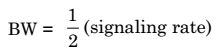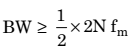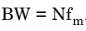### Short Questions and Answers on Multiplexing Techniques

Q.1. What do you mean by Multiplexing?

Ans. Multiplexing may be defined as a technique which allows many users to share a common communication channel simultaneously. There are two major types of multiplexing techniques. They are as under: (i) Frequency division multiplexing (FDM), (ii) Time division multiplexing (TDM).

Q.2. Explain Frequency Division Multiplexing (FDM).

Ans. This technique permits a fixed frequency band to every user in the complete channel bandwidth. Such frequency slot is allotted continuously to that user. As an example, consider that the channel bandwidth is 1 MHz. Let there be ten users, each requiring upto 100 kHz bandwidth. Then the complete channel bandwidth of 1 MHz can be divided into ten frequency bands, i.e., each of 100 kHz and every user can be allotted one independent frequency band. This technique is known as Frequency Division Multiplexing (FDM).

Q.3. What is Transmission Bandwidth of a PAM/TDM Channel?

Ans. The minimum transmission bandwidth of a PAM-TDM channel is given by:Therefore, minimum transmission bandwidthHence, minimum transmission bandwidthQ.4. What is Crosstalk in PAM/TDM System?

Ans. Crosstalk basically means interference between the adjacent TDM channels. Infact, it is the unwanted coupling of information from one channel to the other. The guard time ‘Tg’ is the time spacing introduced between the adjacent TDM channels.

Q.5. Write the Advantages of TDM.

Ans. (i) Full available channel bandwidth can be utilized for each channel.

(ii) Intermodulation distortion is absent.

(iii) TDM circuitry is not very complex.

(iv) The problem of crosstalk is not severe.

Q.6. Write the Disadvantages of TDM.

Ans. (i) Synchronization is essential for proper operation.

(ii) Due to slow narrowband fading, all the TDM channels may get wiped out.

Q.7. Explain the Principle of Digital Multiplexing.

Ans. Digital multiplexing is based on the principle of “interleaving symbols” from two or more digital signals. This is similar to TDM but it does not require the periodic sampling and waveform preservation. The signals which are to be multiplexed may come from digital data sources or from analog sources that have been digitally coded. A digital multiplexer is used to merge the input bits from different sources to form one signal from transmission via a digital communication system. The multiplexed signal consists of source digits interleaved bit by bit or word by word.

The important functions that must be performed by a multiplexer are as under:

(i) To establish a frame. A frame consists of at least one bit from every input.

(ii) A number of unique bits slots within the frame should be assigned to each input.

(iii) To insert control bits for frame identification and synchronization.

(iv) To make allowance for any variations of the input bit rates.

Q.8. How can you classify Digital Multiplexers?

Ans. The various digital sources that are to be multiplexed will have different bit rates. In practice, the bit rate variation poses the most serious design problem and leads to the three categories of multiplexers, as under:

(i) Synchronous multiplexers

(ii) Asynchronous multiplexers

(iii) Quasi-synchronous multiplexers.

Q.9. Write the advantages of Digital Multiplexing.

Ans. (i) Hardware cost reduction due to the use of digital ICs.

(ii) Power cost reduction due to use of regenerative repeaters.

(iii) More flexibility as compared to the analog multiplexers.

Q.10. What is FDM? Where is it used?

Ans. Frequency Division Multiplexing (FDM) is based on the principle of isolating the channels (to be sent simultaneously) in frequency domain. It is used for analog signals such a telephone signals.

Q.11. What is TDM? How is it different from FDM?

Ans. The Time Division Multiplexing (TDM) is based on the principle of ‘time sharing’ the common communication channel. Various TDM signals occupy the same slot in the frequency spectrum.

Q.12. When do you prefer TDM to FDM?

Ans. TDM is preferred when:

(i) The signals are digital

(ii) When there is possibility of wideband fading.

Q.13. When would you prefer FDM to TDM?

Ans. FDM is preferred when:

(i) The signals are analog,

(ii) Synchronization is not to be used

(iii) When there is a possibility of slow narrow band fading.

Q.14. What is meant by signaling rate?

Ans. It is the number of pulses transmitted per second.

Q.15. What is the minimum rate of sending the TDM signals?

Ans. The Nyquist rate.

Q.16. The process of transmitting two or more signals simultaneously on the same medium is called ………..

Ans. Multiplexing.

Q.17. Define the term ‘Frame’ in case of TDM.

Ans. One frame corresponds to one complete revolution of the commutator. It contains one sample from each signal and a marker pulse for synchronization.

Q.18. What is crosstalk? How to reduce it?

Ans. Crosstalk is the interference or information coupling between the adjacent channels (signals) which are being multiplexed. It can be reduced by introducing guardbands (FDM) are guard time (TDM).

Q.19. What type of modulation is used in FDM?

Ans. The FDM is based on the concept of sharing bandwidth of a common communication channel. The modulation can be AM, SSB, FM or PM.

Q.20. Define the FDM Hierarchy.

Ans. The FDM hierarchy are the following differential levels as follows:

Level 1: Basic group – 12 voice channels multiplexed together

Level 2: Super group – upto 5 basic groups

Level 3: Master group – upto 10 super groups Level 4: Jumbo group – upto 6 master groups.

Q.21. Does FDM need synchronization?

Ans. No, FDM does not need synchronization at all. This is its advantage over the TDM.

Q.22. What are the advantages of FDM system?

Ans. They are preferred for the analog signals, easy circuitry, most widely used technology.

Q.23. What are the disadvantages of FDM system?

Ans. FDM needs complex circuit, it suffers from the problem of crosstalk, all the FDM channels suffer due to the wideband fading.